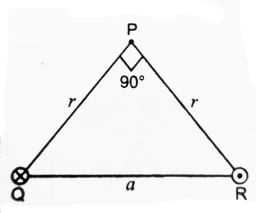# The trapezium

The trapezium is formed by cutting the top of the right-angled isosceles triangle. The base of the trapezium is 10 cm and the top is 5 cm. Find the area of trapezium.

A =  18.75 cm2

### Step-by-step explanation:Did you find an error or inaccuracy? Feel free to write us. Thank you!Tips to related online calculators
Pythagorean theorem is the base for the right triangle calculator.

#### You need to know the following knowledge to solve this word math problem:

We encourage you to watch this tutorial video on this math problem:

## Related math problems and questions:

• Isosceles trapeziumCalculate the area of an isosceles trapezium ABCD if a = 10cm, b = 5cm, c = 4cm.
• Isosceles trapezoidFind the area of an isosceles trapezoid, if the bases are 12 cm and 20 cm, the length of the arm is 16 cm
• R TrapeziumRectangular trapezium has bases 21 and 10 and area 92 cm2. What is its perimeter?
• Isosceles trapezoidThe old father decided to change the top plate of an isosceles-like trapezoid with the basic dimensions of 120 cm and 60 cm, and the shoulder is 50 centimeters long. How much does it pay for a new plate and a square meter worth 17 euros?
• Isosceles triangleCalculate the area of an isosceles triangle, the base of which measures 16 cm and the arms 10 cm.
• Isosceles trapezoidCalculate the area of an isosceles trapezoid whose bases are in the ratio of 4:3; leg b = 13 cm and height = 12 cm.
• Isosceles trapezoidThe lengths of the bases of the isosceles trapezoid are in the ratio 5:3, the arms have a length of 5 cm and height = 4.8 cm. Calculate the circumference and area of a trapezoid.
• ISO trapezoid v2bases of Isosceles trapezoid measured 20 cm and 4 cm and its perimeter is 55 cm. What is the are of a trapezoid?
• Area of iso-trapFind the area of an isosceles trapezoid if the lengths of its bases are 16 cm and 30 cm, and the diagonals are perpendicular to each other.
• IS trapezoidCalculate the length of diagonal u and height v of isosceles trapezoid ABCD, whose bases have lengths a = |AB| = 37 cm, c = |CD| = 29 cm and legs b = d = |BC| = |AD| = 28 cm.
• Isosceles trapezoidIn an isosceles trapezoid KLMN intersection of the diagonals is marked by the letter S. Calculate the area of trapezoid if /KS/: /SM/ = 2:1 and a triangle KSN is 14 cm2.
• TrapeziumThe length of the base and the height size of the base of the trapezium is at ratio 5:3:2, the content area of the trapezium is 128 cm2. Calculate the length of the base and the height of the trapezoid.
• Isosceles trapezoidWhat is the height of an isosceles trapezoid, the base of which has a length of 11 cm and 8 cm and whose legs measure 2.5 cm?
• Isosceles trapezoidCalculate the circumference and the contents of the isosceles trapezoid if you know the size of the bases is 8 and 12 cm and the size of the arms is 5 cm.
• DiagonalThe rectangular ABCD trapeze, whose AD arm is perpendicular to the AB and CD bases, has an area of 15 cm square. Bases have lengths AB = 6cm, CD = 4cm. Calculate the length of the AC diagonal.
• Isosceles trapezoidFind the height in an isosceles trapezoid if the area is 520 cm2 and the base a = 25 cm and c = 14 cm. Calculate the interior angles of the trapezoid.
• Rectangular trapezoidThe ABCD rectangular trapezoid with the AB and CD bases is divided by the diagonal AC into two equilateral rectangular triangles. The length of the diagonal AC is 62cm. Calculate trapezium area in cm square and calculate how many differs perimeters of the